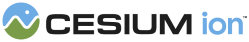#QuarticRealPolynomial

Defines functions for 4th order polynomial functions of one variable with only real coefficients.

### Methods

#### static Cesium.QuarticRealPolynomial.computeDiscriminant(a, b, c, d, e) → number engine/Source/Core/QuarticRealPolynomial.js 23

Provides the discriminant of the quartic equation from the supplied coefficients.
Name Type Description
`a` number The coefficient of the 4th order monomial.
`b` number The coefficient of the 3rd order monomial.
`c` number The coefficient of the 2nd order monomial.
`d` number The coefficient of the 1st order monomial.
`e` number The coefficient of the 0th order monomial.
##### Returns:
The value of the discriminant.

#### static Cesium.QuarticRealPolynomial.computeRealRoots(a, b, c, d, e) → Array.<number> engine/Source/Core/QuarticRealPolynomial.js 273

Provides the real valued roots of the quartic polynomial with the provided coefficients.
Name Type Description
`a` number The coefficient of the 4th order monomial.
`b` number The coefficient of the 3rd order monomial.
`c` number The coefficient of the 2nd order monomial.
`d` number The coefficient of the 1st order monomial.
`e` number The coefficient of the 0th order monomial.
##### Returns:
The real valued roots.
Need help? The fastest way to get answers is from the community and team on the Cesium Forum.